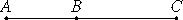# Proposition 39

If two straight lines incommensurable in square which make the sum of the squares on them rational but the rectangle contained by them medial are added together, then the whole straight line is irrational; let it be called major.
X.33

Let two straight lines AB and BC incommensurable in square, and fulfilling the given conditions, be added together.

I say that AC is irrational.Since the rectangle AB by BC is medial, therefore twice the rectangle AB by BC is also medial.

But the sum of the squares on AB and BC is rational, therefore twice the rectangle AB by BC is incommensurable with the sum of the squares on AB and BC, so that the sum of the squares on AB and BC together with twice the rectangle AB by BC, that is, the square on AC, is also incommensurable with the sum of the squares on AB and BC. Therefore the square on AC is irrational, so that AC is also irrational. Let it be called major.

Q.E.D.

## Guide

This proposition is used in X.57, X.63, and X.68.